Gaseous - Thermodynamic - Thermochemistry

# Gaseous - Thermodynamic - Thermochemistry

Test Description

## 60 Questions MCQ Test Mock Test Series for IIT JAM Chemistry | Gaseous - Thermodynamic - Thermochemistry

Gaseous - Thermodynamic - Thermochemistry for Chemistry 2023 is part of Mock Test Series for IIT JAM Chemistry preparation. The Gaseous - Thermodynamic - Thermochemistry questions and answers have been prepared according to the Chemistry exam syllabus.The Gaseous - Thermodynamic - Thermochemistry MCQs are made for Chemistry 2023 Exam. Find important definitions, questions, notes, meanings, examples, exercises, MCQs and online tests for Gaseous - Thermodynamic - Thermochemistry below.
Solutions of Gaseous - Thermodynamic - Thermochemistry questions in English are available as part of our Mock Test Series for IIT JAM Chemistry for Chemistry & Gaseous - Thermodynamic - Thermochemistry solutions in Hindi for Mock Test Series for IIT JAM Chemistry course. Download more important topics, notes, lectures and mock test series for Chemistry Exam by signing up for free. Attempt Gaseous - Thermodynamic - Thermochemistry | 60 questions in 180 minutes | Mock test for Chemistry preparation | Free important questions MCQ to study Mock Test Series for IIT JAM Chemistry for Chemistry Exam | Download free PDF with solutions
 1 Crore+ students have signed up on EduRev. Have you?
Gaseous - Thermodynamic - Thermochemistry - Question 1

### For one mole of an ideal gas, increasing the temperature from 10°C to 20°C ______.

Gaseous - Thermodynamic - Thermochemistry - Question 2

### With increase of pressure, the mean free path:

Gaseous - Thermodynamic - Thermochemistry - Question 3

### A gas is liquefied:

Gaseous - Thermodynamic - Thermochemistry - Question 4

At what temperature the RMS velocity of SO2 be same as that of O2 at 303 K

Gaseous - Thermodynamic - Thermochemistry - Question 5

An ideal gas obeying kinetic theory of gases can be liquefied if:

Gaseous - Thermodynamic - Thermochemistry - Question 6

For the process
H2O(l) → H2O(g)
At T = 100°C and 1 atmosphere pressure, the correct choice is:

Gaseous - Thermodynamic - Thermochemistry - Question 7

One mole of a monoatomic ideal gas expands adiabatically at initial temperature T against a constant external pressure of 1 atm from 1L to 3L. The final temperature of the gas is:

Gaseous - Thermodynamic - Thermochemistry - Question 8

The enthalpies of combustion of carbon and carbon monoxide are –393.5 and –283 kJ mol–1, respectively. The enthalpy of formation of carbon monoxide per mole is:

Gaseous - Thermodynamic - Thermochemistry - Question 9

The Maxwell's relationship derived from the equation dG = VdP – SdT is:

Gaseous - Thermodynamic - Thermochemistry - Question 10

For a closed system consisting of a reaction N2O4(g) → 2NO2(g), the pressure:

Gaseous - Thermodynamic - Thermochemistry - Question 11

One mole of an ideal gas is put through a series of changes as shown in the graph in which A, B, C mark the three stages of system. Calculate the pressure at three stages of the system & provide the sum value (PA + PB + PC)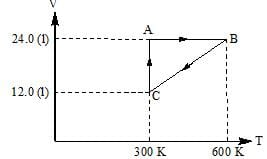Gaseous - Thermodynamic - Thermochemistry - Question 12

If at 298 K the bond energies of C—H, C—C, C=C and H—H are, respectively, 414, 347, 615 and 435 kJ mol–1, the value of enthalpy change for the reaction CH2=CH2(g) + H2(g) → H3C—CH3(g) at 298 K will be:

Gaseous - Thermodynamic - Thermochemistry - Question 13

2 mole 'He' is mixed with 2gm of H2. The molar heat capacity at constant pressure for mixture is:

Gaseous - Thermodynamic - Thermochemistry - Question 14

An ideal gas is allowed to expand both reversibly and irreversibly in an isolated system. If Ti is the initial temperature and Tf is the final temperature, which of the following statement is correct:

Gaseous - Thermodynamic - Thermochemistry - Question 15

An inventor claims to have constructed an engine that has an efficiency of 75% when operated between the boiling and freezing points of water. Which of the following is true?

Gaseous - Thermodynamic - Thermochemistry - Question 16

If C1, C2, C3........ represent the speeds of n1, n2, n3....... molecules, then the root mean square speed is:

Gaseous - Thermodynamic - Thermochemistry - Question 17

A bubble of air is underwater at temperature 15°C and the pressure 1.5 bar. If the bubble rises to the surface where the temperature is 25°C and the pressure is 1.0 bar, what will happen to the volume of the bubble?​

Gaseous - Thermodynamic - Thermochemistry - Question 18

0.5 mole of each of H2, SO2 and CH4 are kept in a container. A hole was made in the container. After 3 hours, the order of partial pressures in the container will be:

Gaseous - Thermodynamic - Thermochemistry - Question 19

Volume of air that will be expelled from a vessel of 300 cm3 when it is heated from 27oC o 37oC at the same pressure will be

Gaseous - Thermodynamic - Thermochemistry - Question 20

Two separate bulbs contain ideal gases A and B. The density of gas A is twice that of gas B. The molecular mass of A is half that of gas B. The two gases are at the same temperature. The ratio of the pressure of A to that of gas B is:

Gaseous - Thermodynamic - Thermochemistry - Question 21

The molar entropy of crystalline CO at absolute zero is:

Gaseous - Thermodynamic - Thermochemistry - Question 22

The plot that describes a Carnot cycle is:

Gaseous - Thermodynamic - Thermochemistry - Question 23

The rate of evaporation of a liquid is always faster at a higher temperature because:

Gaseous - Thermodynamic - Thermochemistry - Question 24

For the process,
1 Ar (300 K, 1 bar) → 1Ar (200 K, 10 bar) assuming ideal gas behaviour, the change in molar entropy is:

Gaseous - Thermodynamic - Thermochemistry - Question 25

For the reaction, N2(g) + 3H2(g) → 2NH3(g)
​Compute the entropy change (in J/K/mol) for the process and comment on the sign of the property.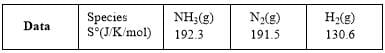Gaseous - Thermodynamic - Thermochemistry - Question 26

For the reaction,
2Cl(g) → Cl2(g)
The thermodynamic properties

Gaseous - Thermodynamic - Thermochemistry - Question 27

The standard enthalpies of formation of CO2(g), H2O(l) and glucose(s) at 25°C are –400 kJ mol–1,
–300 kJ mol–1 and –1300 kJ mol–1, respectively. The standard enthalpy of combustion per gram of glucose at 25°C is:

Gaseous - Thermodynamic - Thermochemistry - Question 28

For an ideal gas, which of the following is true:

Gaseous - Thermodynamic - Thermochemistry - Question 29

Assuming that water vapour is an ideal gas, the internal energy change (∆U) when 1 mol of water is vaporized at 1 bar pressure and 100°C will be (given that molar enthalpy of vaporisation of water at 1 bar and 373 K is 41 kJ mol–1 K–1)

Gaseous - Thermodynamic - Thermochemistry - Question 30

For a system of constant composition, the pressure (P) is given by:

*Multiple options can be correct
Gaseous - Thermodynamic - Thermochemistry - Question 31

Identify the intensive quantities from the following:

*Multiple options can be correct
Gaseous - Thermodynamic - Thermochemistry - Question 32

Among the following which are/is state function(s):

*Multiple options can be correct
Gaseous - Thermodynamic - Thermochemistry - Question 33

Select the correct statements for ideal gases:

*Multiple options can be correct
Gaseous - Thermodynamic - Thermochemistry - Question 34

The incorrect expression among the following is:

Gaseous - Thermodynamic - Thermochemistry - Question 35

Indicate which one of the following relations is not correct:

*Multiple options can be correct
Gaseous - Thermodynamic - Thermochemistry - Question 36

The species which by definition has zero standard molar enthalpy of formation at 298 K is:

*Multiple options can be correct
Gaseous - Thermodynamic - Thermochemistry - Question 37

For an ideal gas at 300 K:

Gaseous - Thermodynamic - Thermochemistry - Question 38

An ideal gas obeying kinetic theory of gases can be liquefied if:

*Multiple options can be correct
Gaseous - Thermodynamic - Thermochemistry - Question 39

Sponteneous adsorption of a gas on solid surface is an exothermic process because

*Multiple options can be correct
Gaseous - Thermodynamic - Thermochemistry - Question 40

Indicate the correct statement for a 1-L sample of N2(g) and CO2(g) at 298 K and 1 atm pressure:

*Answer can only contain numeric values
Gaseous - Thermodynamic - Thermochemistry - Question 41

A gas expands against a variable external pressure given by p = 10/V atm, where V is the volume at each stage of expansion. In expanding from 10 L to 100 L, the gas undergoes a change in internal energy ∆U = 418 J. How much heat (kJ) has been absorbed? [rounded up to two decimal places]

*Answer can only contain numeric values
Gaseous - Thermodynamic - Thermochemistry - Question 42

Calculate the work done (J) when 1 mole of zinc dissolves in hydrochloric acid at 273 K in a closed beaker at 300 K [Volume at STP = 22.4 L, rounded up to two decimal places]:

*Answer can only contain numeric values
Gaseous - Thermodynamic - Thermochemistry - Question 43

Calculate the enthalpy change (calmol–1) when 36 g of water is heated from 27°C to 37°C at 1 atm pressure. The molar heat capacity of water (18.1 cal K–1mol–1) remains constant in this temperature range: [rounded up to two decimal places]

*Answer can only contain numeric values
Gaseous - Thermodynamic - Thermochemistry - Question 44

Given the following thermo chemical equations: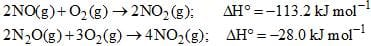Calculate ∆H°(kJ mol–1) for this reaction: N2O(g) + 1/2 O2(g) → 2NO(g) [rounded up to two decimal places]

*Answer can only contain numeric values
Gaseous - Thermodynamic - Thermochemistry - Question 45

Acetone, the solvent usually found in nail polish remover and has the structural formula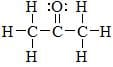Approximately how much energy (kJ) would be released during the formation of the bonds in one mole of acetone molecules? [In the multiple of 103, rounded up to two decimal places]
Given: C—H = 412 kJ/mol; C—C = 348; C=O = 743.

*Answer can only contain numeric values
Gaseous - Thermodynamic - Thermochemistry - Question 46

Diborane is a potential rocket fuel which undergoes combustion according to the reaction,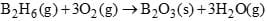From the follwoing data, calculate the enthalpy change (kJ mol–1) for the combustion of diborane: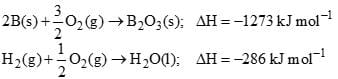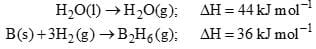*Answer can only contain numeric values
Gaseous - Thermodynamic - Thermochemistry - Question 47

A gas is found to have a formula [CO]x. If its vapor density is 70, the value of x is:

*Answer can only contain numeric values
Gaseous - Thermodynamic - Thermochemistry - Question 48

2g of gas X are introduced into an evacuated flask kept at 25oC. The pressure is found to be 1atm. If 3g of another gas ‘Y’ are added to same flask, the total pressure becomes 1.5 atm. Assuming that ideal behavior, the molecular mass ratio of Mx and My is:

*Answer can only contain numeric values
Gaseous - Thermodynamic - Thermochemistry - Question 49

The density of a gas at 27°C and 1 atm is d. Pressure remaining constant at which temperature(K) will its density become 0.75 d: [rounded up to two decimal places]

*Answer can only contain numeric values
Gaseous - Thermodynamic - Thermochemistry - Question 50

Pressure exerted by 1 mole of methane in a 0.25 litre container at 300 K using van der Waal's equation (given a = 2.253 atml2mol–2, b = 0.0428 litmol–1) is (in atm): [rounded up to two decimal places]

*Answer can only contain numeric values
Gaseous - Thermodynamic - Thermochemistry - Question 51

Find the maximum work done (cal) when 0.5 mol of a gas expands isothermally and reversibly from a volume of 2L to 5L at 27°C and calculate the change in internal energy if 200 cal of heat is absrobed? [rounded up to two decimal places]

*Answer can only contain numeric values
Gaseous - Thermodynamic - Thermochemistry - Question 52

For the following reaction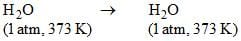∆H = 40 kJ/mol
Cp(H2O, l) = 40 J/mol K
Cp(H2O, g) = 30 J/mol K
Then calculate ∆G(J/mol) at 1 atm, 373 K:

*Answer can only contain numeric values
Gaseous - Thermodynamic - Thermochemistry - Question 53

For the reaction,

2CO + O2 → 2CO2;   ΔH = -560KJ
Two moles of CO and one mole of O2 are taken in a container of volume 1L. They completely form two moles of CO2, the gases deviated appreciably from ideal behavior. If the pressure in the vessel changes from 70 to 40 atm, find the magnitude (kJ) of ∆U at 500 K. (1L atm = 0.1 kJ) [rounded up to two decimal places]

*Answer can only contain numeric values
Gaseous - Thermodynamic - Thermochemistry - Question 54

If 22 g of CO2, showing ideal behaviour, isothermally and reversibly from 2.5 L to 7.5 L at 27°C, then calculate the work done (J) and heat absorbed: [rounded up to two decimal places]

*Answer can only contain numeric values
Gaseous - Thermodynamic - Thermochemistry - Question 55

One mole of an ideal gas is subjected to a change from 10 atm to 1 atm at 300 K. Calculate (in JK-1 rounded up to three decimal places) ∆Ssurrounding = ? [R = 8.314 JK-1mol-1]

*Answer can only contain numeric values
Gaseous - Thermodynamic - Thermochemistry - Question 56

What is the boiling point(°C) of a liquid with entropy of vaporization 68.3 JK–1mol–1 and enthalpy of vaporization 35.65 kJ mol–1: [rounded up to two decimal places]

*Answer can only contain numeric values
Gaseous - Thermodynamic - Thermochemistry - Question 57

2 mol of an ideal gas expanded isothermally and reversibly from 1L to 10L at 300 K. What is the enthalpy change(kJ)?

*Answer can only contain numeric values
Gaseous - Thermodynamic - Thermochemistry - Question 58

Containers A and B have same gases. Pressure, volume and temperature of A are all twice that of B, then the ratio of number of molecules of A and B are:

*Answer can only contain numeric values
Gaseous - Thermodynamic - Thermochemistry - Question 59

At identical temp and pressure the rate of diffusion of hydrogen gas is 5.19 times that of hydrocarbon having molecular formula CnH2n-2. What is value of ‘n’ ?

*Answer can only contain numeric values
Gaseous - Thermodynamic - Thermochemistry - Question 60

For one mole of a Vander Waals gas when b = 0 and T = 300 K, the PV vs 1/V plot is shown below. The value of the van der Waals constant a (atm. litre2mol–2) is: [rounded up to first decimal place]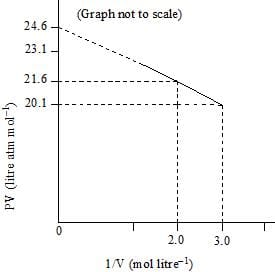## Mock Test Series for IIT JAM Chemistry

2 docs|25 tests
 Use Code STAYHOME200 and get INR 200 additional OFF Use Coupon Code
Information about Gaseous - Thermodynamic - Thermochemistry Page
In this test you can find the Exam questions for Gaseous - Thermodynamic - Thermochemistry solved & explained in the simplest way possible. Besides giving Questions and answers for Gaseous - Thermodynamic - Thermochemistry, EduRev gives you an ample number of Online tests for practice

2 docs|25 tests